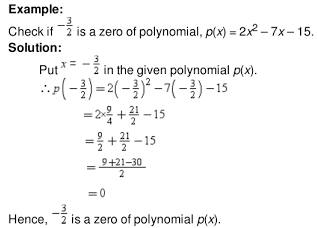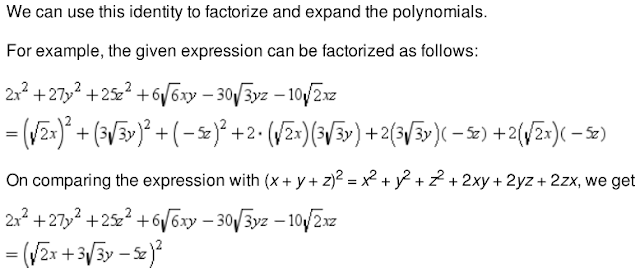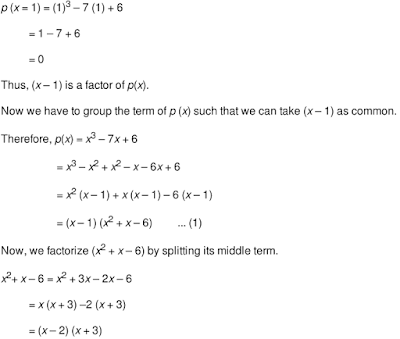## Revision Notes of Ch 2 Polynomials Class 9th Math

Topics in the Chapter

• Polynomials
• Classification of polynomials on the basis of number of terms
• Degree of a Polynomial
• Values of polynomials at different points
• Zeroes of a polynomial
• Identity: (x + y + z)2 = x2 + y2 + z2 + 2xy + 2yz + 2zx
• Remainder Theorem
• Factor Theorem
• Identity: x3 + y3 + z3 – 3xyz = (x + y + z)(x2 + y2 + z2 – xy – yz – zx)

Polynomials

→ An algebraic expression in which the exponents of the variables are non-negative integers
are called polynomials.

For example: 3x4 + 2x3 + x + 9, 3x2 etc.

Constant polynomial

→ A constant polynomial is of the form p(x) = k, where k is a real number.
For example, –9, 10, 0 are constant polynomials.

Zero polynomial

A constant polynomial ‘0’ is called zero polynomial.

General form of a polynomial

A polynomial of the form where are p(x) = anxn + an-1xn-1 + .... + a1x + a0x where a0, a1... ar, are constant and an≠ 0.
Here, a0, a1... an are the respective coefficients of x0,x1, x2 ... xn and n is the power of
the variable x.
anxn + an-1xn-1 - a0 and are called the terms of p(x).

Classification of polynomials on the basis of number of terms

• A polynomial having one term is called a monomial. e.g. 3x, 25t3 etc.

• A polynomial having one term is called a monomial. e.g. 2t-6, 3x4 +2x etc.

• A polynomial having one term is called a monomial. e.g. 3x4 + 8x + 7 etc.

Degree of a Polynomial

• The degree of a polynomial is the highest exponent of the variable of the polynomial.
For example, the degree of polynomial 3x4 + 2x3 + x + 9 is 4.

• The degree of a term of a polynomial is the value of the exponent of the term.

Classification of polynomial according to their degrees

• A polynomial of degree one is called a linear polynomial e.g. 3x+2, 4x, x+9.

• A polynomial of degree is called a quadratic polynomial. e.g. x2+ 9, 3x2 + 4x + 6

• A polynomial of degree three is called a cubic polynomial e.g. 10x3+ 3, 9x3

Note: The degree of a non-zero constant polynomial is zero and the degree of a zero polynomial is not defined.

Values of polynomials at different points

• A polynomial is made up of constants and variables. Hence, the value of the polynomial changes
as the value of the variable in the polynomial changes.
• Thus, for the different values of the variable x, we get different values of the polynomial.Zeroes of a polynomial

• A real number a is said to be the zero of polynomial p(x) if p(a) = 0, In this case, a is also called the root of the equation p(x) = 0.
Note:
• The maximum number of roots of a polynomial is less than or equal to the degree of the polynomial.
• A non-zero constant polynomial has no zeroes.
• A polynomial can have more than one zero.Division of a polynomial by a monomial using long division method

Example: Divide x4 -2x3- 2x2+7x-15 by x-2Identity: (x + y + z)2 = x2 + y2 + z2 + 2xy + 2yz + 2zxIdentities: (x + y)3 = x3 + y3 + 3xy(x+y) and (x - y)3 = x3 - y3 - 3xy(x-y)

Other ways to represent these identities are:
• x3+ y3 = (x+y)3 - 3xy(x+y)
• x3+ y3 = (x+y)(x2-xy+y2)
• x3- y3 = (x-y)3 + 3xy(x-y)
• x3- y3 = (x-y)(x2+xy+y2)

Example: Expand (3x+2y)3 - (3x-2y)3Remainder Theorem

If p(x) is a polynomial of degree greater than or equal to one and a is any real number then if p(x) is divided by the linear polynomial x-a, the remainder is p(a).

Example:
Find the remainder when x5 -x2 +5 is divided by x-2.
Solution:
p(x) = x5 -x2 +5
The zero of x-2 is 2.
p(2) = 25 -22 +5 = 32-4+5 = 33
Therefore, by remainder theorem, the remainder is 33.

Factor Theorem

If p(x) is a polynomial of degree n≥1 and a is any real number, then
• x-a is a factor of p(x), if p(a)=0.
• p(a) = 0, if (x-a) is a factor of p(x).

Example:
Determine whether x +3 is a factor of x3+5x2+5x-3.
Solution
The zero of x+3 is -3.
Let p(x) = x3+5x2+5x-3
p(-3) = (3)3+5(-3)2 + 5(-3)-3
= -27+45-15-3
= -45 + 45
= 0
Therefore, by factor theorem, x + 3 is the factor of p(x).
Factorisation of quadratic polynomials of the form ax2+bx+c can be done using Factor
theorem and splitting the middle term.

Factorize x2– 7x + 10 using the factor theorem

Let p(x) = x2– 7x + 10
The constant term is 0 and its factors are ±1, ±2, ±5 and ±1O.
Let us check the value of the polynomial for each of these factors of 10.
p(1) = 12– 7×1 + 10 = 1-7+10 = 4 ≠ 0
Hence, x-1 is not a factor of p(x),
p(2) = 22– 7×2 + 10 = 4-14+10 = 0
Hence, x -2 is a factor of p(x).
p(5) = 52- 7×5 +10 = 25-35+10 = 0
Hence, x -5 is a factor of p(x).
We know that a quadratic polynomial can have a maximum of two factors. We have obtained the two factors of the given polynomial, which are x-2 and x-5.
Thus, we can write the given polynomial as:
Let p(x) = x2– 7x + 10 = (x-2) (x-5)

Factorize 2x2 – 11x + 15 by splitting the middle term.

The given polynomial is 2x2– 11x + 15
Here, a = 2×15 = 30. The middle term is -11. Therefore, we have split -11 into two numbers such that their product is 30 and their sum is -11. These numbers are -5 and -6 [As (-5)+ (-6) = -11 and -5 × -6 = 30]
Thus, we have:
2x2– 11x + 15 = 2x2– 5x - 6x + 15
= x(2x- 5) -3 (2x- 5)
= (x-3) (2x-5)

Note: A quadratic polynomial can have a maximum of two factors.

• Factorisation of cubic polynomials of the form ax3+ bx2+ cx can be done using factor
theorem and hit and trial method.

A cubic polynomial can have a maximum of three linear factors. So, by knowing one of these
factors, we can reduce it to a quadratic polynomial.
Thus, to factorize a cubic polynomial, we first find a factor by the hit and trial method or by using the factor theorem, and then reduce the cubic polynomial into a quadratic polynomial and it is then solved further.

Factorise p(x) = x3 – 7x + 6

The constant term is 6.
The factors of 6 are ±1, ±2, ±3 and ±6.
Let x = 1From equation (1), we get
p(x) = (x – 1) (x – 2) (x + 3)
Hence, factors of polynomial p(x) are (x – 1), (x – 2) and (x + 3).

Identity: x3 + y3 + z3 – 3xyz = (x + y + z)(x2 + y2 + z2 – xy – yz – zx)